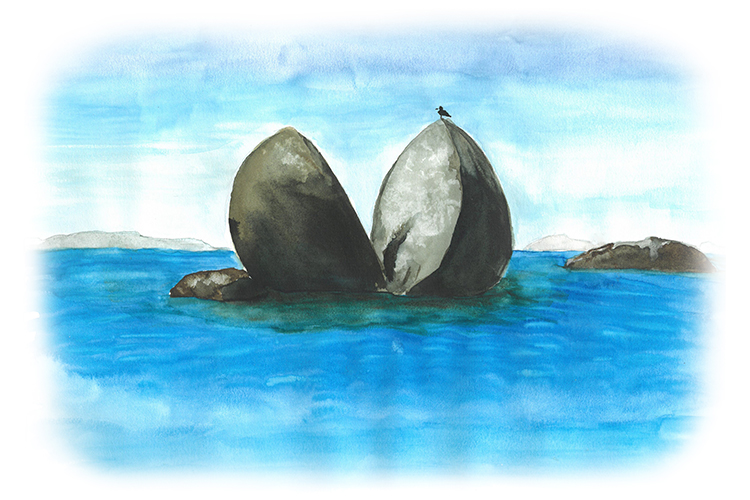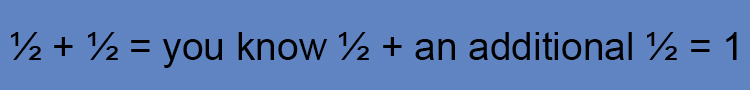# Adding Fractions = Think 1/2

Whenever you see the word fractions think of 1/2The fractured (fraction) rock was split in half ( 1/2 )

To remember how to calculate fractions always think of a 1/2 and work it out from there:From this you can use logic and ask yourself how do you get from 1/2+1/2 to equal 1

It must be:  (1+1)/2=2/2=1